# GED Math : Faces and Surface Area

## Example Questions

1 2 4 Next →

### Example Question #157 : 3 Dimensional Geometry

You have a cube with surface area of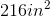.

What is the area of one face of the cube?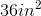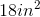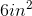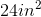Explanation:

You have a cube with surface area of.

What is the area of one face of the cube?

To find the surface area of a cube, we use the following formula: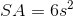Where s squared gives us the area of one face. We multiply by 6 because there are six faces.

So, we can rewrite our above formula to get: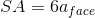So, if we plug in our given surface area, we can find the area of one face.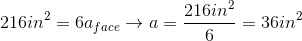### Example Question #158 : 3 Dimensional Geometry

A paint factory uses cylindrical paint cans that have a radius ofinches and a height ofinches. If the factory can make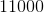cubic inches of paint a day, how many complete cans can the factory fill in one day?Explanation:

Start by finding the volume of the paint can. Recall how to find the volume of a cylinder: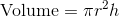, whereis the radius andis the height.

Plug in the given radius and height to find the volume of the paint can.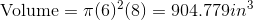Now, divide the total volume of paint made by the factory in a day by the volume of an individual paint can to determine how many cans will be filled.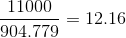Since the question asks for the number of complete cans that will be filled, the factory can only fillcans a day.

### Example Question #31 : Faces And Surface Area

Consider a tube which is 3 ft wide and 18 ft long.

Find the surface area of the largest sphere which could fit within the tube described above.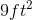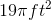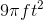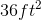Explanation:

Consider a tube which is 3 ft wide and 18 ft long.

Find the surface area of the largest sphere which could fit within the tube described above.

Okay, so we need to find the surface area of a sphere. To do so, we need the following formula: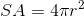Now, you're probably thinking, "How do we find our radius?" Well, we need to look at our tube.

The largest sphere which will fit within the tube is the same thing as a sphere with diameter equal to the tube's diameter.

In this case, the diameter of the tube is 3 ft. This means our sphere's diameter is also 3 feet.

If our diameter is 3 ft, then our radius is half of that- 1.5 ft.

So, with a 1.5 ft radius, our surface area becomes: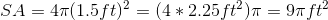So, leaving our answer in terms of pi, we get:### Example Question #159 : 3 Dimensional Geometry

Find the surface area of a rectangular prism with the following dimensions: 6 ft by 12 ft by 4 ft.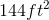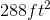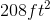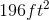Explanation:

Find the surface area of a rectangular prism with the following dimensions: 6 ft by 12 ft by 4 ft.

To find the surface area of a rectangular prism, we essentially need to add up the area of all the sides. To do so, use the following formula: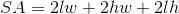Where l, w, and h are our length, height, and width.

We simply need to plug in our given measurements and solve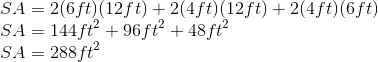So, our surface area is1 2 4 Next →

### All GED Math Resources